# MTA Use Case

#### 2022-06-03

This vignette describes a typical use case of the `MTA` package with a concrete example: the analysis of income inequalities in the Greater Paris Metropolitan Area. This example plays all the functions of the MTA package with appropriate visualizations (plots, maps).

# 1. Introducing case-study and MTA

## 1.1 Multiscalar Territorial Analysis for Policy Study

Beginning in the MTA conceptual framework, several issues must be considered: the study area, the territorial hierarchy and the selected indicator.

• Metropolis of Greater Paris (MGP) : a study area making sense in a political context.
The MGP has been created the 1st January 2016 after a preparatory mission and aims at finding solutions to improve and develop the potential of the Paris metropolitan area to be more present at international level (following the initiatives that took place in London, New-York or Tokyo). The official website (last visit: 2022-06) of this infrastructure provides all the details of this new level of governance. Namely, this spatial planning project proposes to “develop a stronger solidarity between territories, reduce territorial inequalities and propose a rebalancing in term of access to household, employment, training, services and amenities”. For this emerging zoning of spatial planning, the need for indicators and analysis of territorial inequalities is high. The MTA methodology seems to be particularly adapted to propose territorial evidences in this context.

• From the IRIS to the MGP, a lot of hierarchical territorial divisions are available.
The lowest territorial level existing in France associated with public statistical information is IRIS (Ilots Regroupés pour l’Information Statistique). The reference size targeted for each IRIS is 2000 inhabitants (last visit: 2022-06). Each municipality over 10 000 inhabitants and a high proportion of municipalities between 5 000 and 10 000 inhabitants are split in IRIS. It is possible to merge these IRIS in municipalities (36 000 territorial units in France). These municipalities can also be aggregated in Établissements Publics Territoriaux(equivalent to Établissement de Coopération intercommunale, EPCI) or in départements. Each territorial context has specific competences in spatial planning term. Thus, the MGP is constituted by a large number of territorial divisions. It allows the calculation of a high number of deviations according to these territorial contexts. This analysis is mainly focused on municipalities and Établissements Publics Territoriaux (EPT) but could be extended to other territorial levels.

• Indicators relevant at this scale of analysis.
The chosen indicator (average income tax reference per households) is one of the key drivers of spatial planning policies at local level in France. It is one of the indicators considered relevant for city policy (Politique de la Ville, last visit: 2022-06).

This vignette proposes some concrete outputs for improving the knowledge on income inequalities and proposing solutions for a better geographical distribution of wealth in the MGP area. Relevant statistics will be computed using MTA functionalities and plotted on graphics and maps.

## 1.2 Dataset and complementary Packages

The example dataset, `GrandParisMetropole` includes all the required information to create

• com is a sf object. It includes the geometries, identifiers (DEPCOM) and names (LIBCOM) of the 150 MGP municipalities identifiers and names of the EPT of belonging of each municipality (EPT and LIB_EPT), identifier of the département of belonging of each municipality (DEP) and the statistical indicators for the multiscalar inequality analysis, the numerator (amount of income tax references in Euros, INC) and the denominator (number of tax households, TH) (see ?com).

• EPT is a sf object. It includes the geometries of the 12 EPT of the MGP (see ?EPT).

• cardist is a matrix including the a time distance matrix between municipalities computed using `osrm` package (see ?cardist).

Two additional packages are used: `mapsf` for thematic mapping purposes and `ineq` for computing inequality indexes and Lorenz Curve Plot.

``````# load packages
library(sf)``````
``## Linking to GEOS 3.9.0, GDAL 3.2.2, PROJ 7.2.1; sf_use_s2() is TRUE``
``````library(MTA)
library(mapsf)
library(ineq)

com <- st_read(system.file("metroparis.gpkg", package = "MTA"),
layer = "com", quiet = TRUE)
ept <- st_read(system.file("metroparis.gpkg", package = "MTA"),
layer = "ept", quiet = TRUE)

# set row names to municipalities names
row.names(com) <- com\$LIBCOM``````

## 1.3 Context Maps

Context maps highlight the territorial organization of the study area and provide some first insights regarding the spatial patterns introduced by the indicator used for the analysis.

### 1.3.1 Study Area

The 150 municipalities of the MGP belongs to 12 intermediate territorial divisions: the Établissements Publics Territoriaux (EPT). This territorial zoning follows approximately the delineation of the départements : Seine-Saint-Denis (in blue on the map below); Paris (in purple); Hauts-de-Seine (in green) and _Val-de-Marine (in orange).

``````# label / colors management
LIBEPT <- c("Paris", "Est Ensemble", "Grand-Paris Est",
"Territoire des aeroports", "Plaine Commune", "Boucle Nord 92",
"La Defense","Grand Paris Sud Ouest", "Sud Hauts-de-Seine",
"Val de Bievres - Seine Amond - Grand Orly",
"Plaine Centrale - Haut Val-de-Marne - Plateau Briard",
"Association des Communes de l'Est Parisien")

# colors
cols <- c("#cfcfcf", # Grey(Paris)
"#92C8E0", "#7BB6D3", "#64A4C5", "#458DB3", # Blues (dept 93)
"#A6CC99", "#8CBB80", "#71A966", "#4E9345", # Greens (dept 92)
"#F38F84", "#EF6860", "#EA3531") # Reds (dept 94)

colEpt <- data.frame(LIBEPT, cols)

# zoning
mf_map(x = com, var="LIBEPT", type = "typo",
val_order = LIBEPT, pal = cols, lwd = 0.2, border = "white",
leg_pos = "left", leg_title = "EPT of belonging")

mf_map(ept, col = NA, border = "black", add = TRUE)

# layout
mf_layout(title = "Territorial Zoning of the MGP",
credits = paste0("Sources : GEOFLA® 2015 v2.1, Apur, impots.gouv.fr",
"\nRonan Ysebaert, RIATE, 2021"))``````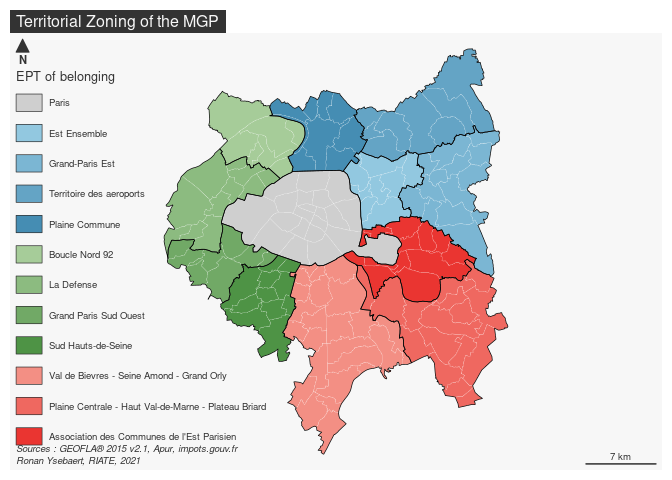### 1.3.2 Map numerator and denominator

The numerator (number of tax households) and the denominator (total amount of income tax references) building the reference ratio (average income per tax households) are firstly plotted.

``````# layout
opar <- par(mfrow = c(1, 2))

# numerator map
com\$INCM <- com\$INC / 1000000
mf_map(com,  col = "peachpuff",  border = NA)
mf_map(ept,  col = NA,  border = "black", add = TRUE)

mf_map(x = com, var = "INCM", type = "prop",
col =  "#F6533A", inches = 0.15, border = "white",
leg_pos = "topleft", leg_val_rnd =  0,
leg_title = "Amount of income taxe reference\n(millions of euros)")

# layout
mf_layout(title = "Numerator - Amount of income tax reference",
credits = paste0("Sources : GEOFLA® 2015 v2.1, Apur, impots.gouv.fr",
"\nRonan Ysebaert, RIATE, 2021"),
arrow = FALSE)

# denominator map
mf_map(com,  col = "peachpuff",  border = NA)
mf_map(ept,  col = NA,  border = "black", add = TRUE)

mf_map(x = com, var = "TH", type = "prop",
col =  "#515FAA", inches = 0.15, border = "white",
leg_pos = "topleft", leg_val_rnd =  -2,
leg_title = "Number of tax households", add = TRUE)

# layout
mf_layout(title = "Denominator - Tax households",
credits = paste0("Sources : GEOFLA® 2015 v2.1, Apur, impots.gouv.fr",
"\nRonan Ysebaert, RIATE, 2021"),
arrow = FALSE)
par(opar)``````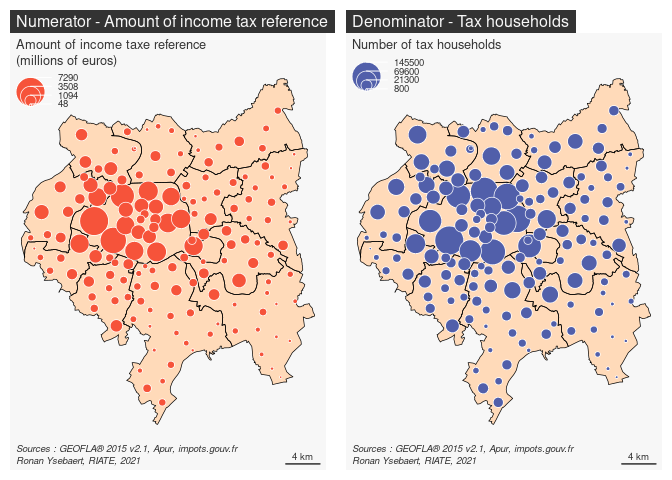Without surprise, the highest amounts of tax households and income are located in the central area of the MGP (Parisian Arrondissements). That being said, these two maps suggest an unequal distribution of income when looking at the distribution of population in Paris suburbs.

### 1.3.3 Ratio

``````# ratio
com\$ratio <- com\$INC / com\$TH

# ratio map
mf_map(x = com, var = "ratio", type = "choro",
breaks = c(min(com\$ratio, na.rm = TRUE),
20000, 30000, 40000, 50000, 60000,
max(com\$ratio, na.rm = TRUE)),
pal = c("#FCDACA", "#F6A599", "#F07168", "#E92B28", "#C70003", "#7C000C"),
border = "white", lwd = 0.2, leg_pos = "topleft", leg_val_rnd = 0,
leg_title = paste0("Average amount of income tax",
"\nreference per households\n(in euros)"))

# EPT borders
mf_map(ept, col = NA, border = "black", add = TRUE)

# Label min and max
mf_label(x = com[which.min(com\$ratio),], var = "LIBCOM", cex = 0.6, font = 2,
halo = TRUE)
mf_label(x = com[which.max(com\$ratio),], var = "LIBCOM", cex = 0.6, font = 2,
halo = TRUE)

# layout
mf_layout(title = "Ratio - Income per tax households, 2013",
credits = paste0("Sources : GEOFLA® 2015 v2.1, Apur, impots.gouv.fr",
"\nRonan Ysebaert, RIATE, 2021"),
arrow = FALSE)``````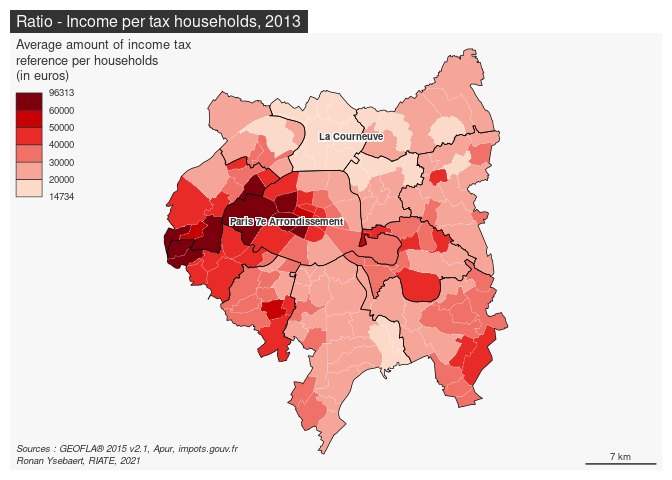The MGP area is characterized by high income inequalities. For the 150 municipalities of this area, the values extend from 14 730 (La Courneuve) to 96 310 euros (Paris_7th Arrondissement). 53 municipalities of the MGP area (35 % of the municipalities) are below the French average, i.e. 25 660 euros. The lagging households are mainly concentrated into the north part of MGP area. Highest values are concentrated in the Western part of Paris and its suburbs.

## 1.4 Introducing the MTA Functions

### 1.4.1 General, territorial and spatial deviations

`MTA` package introduces three contexts to monitor territorial inequalities: the general, the territorial and the spatial deviations.

The global deviation is dedicated to the analysis of inequalities using a value of reference. In this example the global deviation refers to the inequalities existing between each municipality in regard to the whole MGP average.

The territorial deviation consists in measuring the inequalities existing for each basic territorial unit as regards to an intermediate territorial level of reference. In this case-study, it will be the EPT of belonging of each municipality. It implies to include beforehand in the input dataset a territorial level of belong for each territorial unit.

The spatial deviation is a measure of inequalities taking into account the neighborhood as a reference. It allows to measure the deviation existing between basic territorial units using three possible parameters : the territorial contiguity (order 1, 2 or n), the spatial neighborhood (territorial units located at less than X kilometers) or functional distances (territorial units located at less than Y minutes by road for instance). In `MTA`, territorial contiguity and spatial neighborhood measures are directly calculated. Functional distances must be uploaded separately through a dataframe structured with the following fields : id1, id2, distance measure. For this case-study, the contiguity criteria (order 1) will be used for the calculation of the spatial deviation. It gives a good proxy of proximity relationships according to the size and the homogeneity of the municipalities of the MGP area. Other measures could be also adapted, such as time-distances by road (municipalities located at less than 15 minutes by car) or by public transportation.

### 1.4.2 Relative and absolute deviations

In MTA, two methods are implemented to measure statistical differences to a given context of reference: a relative deviation and an absolute deviation. In `MTA`, each indicator is considered as a ratio defined by a numerator (GDP for instance) divided by a denominator (population for instance).

The relative deviation states the position of each region as regards to a reference context. It is based on the following calculation: Relative deviation (Region i) = 100 * ((Numerator(Region i)/Denominator(Region i)) /(reference ratio)) Territorial units characterized by a context of reference below index 100 are under the average of a given reference context, and reciprocally.

The absolute deviation specifies which process of redistribution should be realized in absolute terms to achieve a perfect equilibrium for the ratio of reference in the global, the territorial or the spatial context. It is calculated as below: Absolute deviation (Region i) = Numerator (Region i) - (reference ratio * denominator (Region i)) In concrete terms, it examines how much amount of the numerator should be moved in order to reach equidistribution, for each territorial unit, taking into account as a reference the selected deviation context value. More generally, absolute deviation must be considered as a statistical tool to discuss on the amplitude of existing territorial inequalities. It is obvious that reaching a perfect equilibrium between territorial units is highly sensitive and is scarcely a policy objective itself. It is nevertheless interesting to consider the amount of money or population affected by the inequality to have in hand a concrete material for leading discussions on this delicate spatial planning issue.

### 1.4.3 Cartography of relative and absolute deviations

In this vignette, color palette proposed for displaying on map the relative deviations are the ones suggested by the HyperAtlas tool (blue palette = under the average; red palette = above the average). But other diverging palettes (green/red, etc.) could be also be used for displaying MTA results on maps.

Absolute deviations are highlighted using proportional circles on maps. It examines which amount of the numerator should be moved to the poorest municipalities to reach equidistribution. Circles displayed in a red means that the territorial unit have to contribute a given amount of numerator to achieve the equilibrium in a given context; and reciprocally blue circles means that the territorial unit have to receive frmo the others.

It is quite easy to reproduce or adapt these maps using the functions proposed by the `mapsf` package.

### 1.4.4 Synthesis

The analysis of the three deviations provides generally a lot of information. The synthesis functions `bidev` and `mst` helps to summarize values of the global, territorial and spatial deviations and allow to answer to some basic and interesting questions:

• Which territorial units are positioned in a favorable/lagging situation for 2 or 3 deviations?
• Which territorial units are positioned in a contradictory situations ? This is especially interesting from a social and policy points of view (NIMBY phenomena, characterizing the opposition by residents to a proposal for a new development because it is close to them).

Moreover, some additional functions are implemented to ease the mapping (`map_bidev` and `map_mst`) or proposing appropriate plots (`plot_bidev` and `plot_mst`) for visualizing these results.

# 2. Relative deviations

This section aims at describing how computing MTA relative deviations and creating appropriate maps and plots.

## 2.1 Global deviation

First of all, each territorial unit is compared to the overall study area average (Métropole du Grand Paris).

The code below takes in entry the numerator (INC) and the denominator (TH) of the `com` object and returns the global deviation indicators using `gdev()` function. The relative deviation (`type = "rel"`) is computed. This indicator is afterwards mapped.

``````# general relative deviation
com\$gdevrel <- gdev(x = com,
var1 = "INC",
var2 = "TH",
type = "rel")

# Colors for deviations
devpal <-  c("#4575B4", "#91BFDB", "#E0F3F8", "#FEE090", "#FC8D59", "#D73027")

# Global deviation mapping
mf_map(x = com, var = "gdevrel", type = "choro",
breaks = c(min(com\$gdevrel, na.rm = TRUE), 67, 91, 100, 125, 150,
max(com\$gdevrel, na.rm = TRUE)),
pal = devpal, border = "white", lwd = 0.2,
leg_pos = "topleft", leg_val_rnd = 0,
leg_title = paste0("Deviation to the global context",
"\n(100 = Metropole du Grand Paris average)"))

# Plot EPT layer
mf_map(ept, col = NA, border = "#1A1A19", lwd = 1, add = TRUE)

# layout
mf_layout(title = "Global deviation - Tax income per households",
credits = paste0("Sources : GEOFLA® 2015 v2.1, Apur, impots.gouv.fr",
"\nRonan Ysebaert, RIATE, 2021"),
arrow = FALSE)``````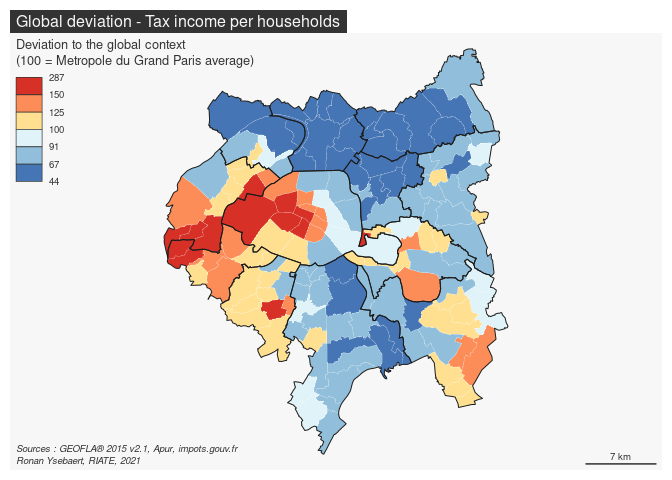The resulting map highlights strong inequalities: First, it is intersting to know that all the municipalities of the EPT of Plaine Commune, Territoire des Aéroports and Est Ensemble are below the average of the MGP area (33 501 euros per households). For the territories of Val de Bièvres and Grand-Paris Est, only three municipalities are above the average of the study area. Reversely, all the municipalities of the Grand-Paris-Sud-Ouest EPT are largely above the average of the MGP area. For the other EPT the situation is mixed, depending of the municipalities.

The `ineq` package proposes some functions to depict global inequalities existing in a study area, such as the Lorenz-curve. The `Lc` function takes in entry the numerator and the denominator and returns a Lorenz Curve plot; inequality indexes take in entry the ratio (numerator / denominator) and returns econometric indexes of inequality.

The code below add some additional graphical parameters to ease the interpretation of this plot.

``````library(ineq)
#  Concentration of X as regards to concentration of Y
Lc.p <- Lc(com\$INC, com\$TH)
Lp <- data.frame(cumX = 100 * Lc.p\$L, cumY = 100 * Lc.p\$p)

# Plot concentrations
opar <- par(mar = c(4,4,1.2,4), xaxs = "i", yaxs = "i", pty = "s")
plot(Lp\$cumY, Lp\$cumX,  type = "l", col = "red", lwd = 2,
panel.first = grid(10,10), xlab = "Households (cumulative percentage)",
ylab = "Income (cumulative percentage)",
cex.axis = 0.8, cex.lab = 0.9, ylim = c(0,100), xlim = c(0,100))

lines(c(0,100), c(0,100), lwd = 2)

xy1 <- Lp[which.min(abs(50 - Lp\$cumX)),]
xy2 <- Lp[which.min(abs(50 - Lp\$cumY)),]

xy <- rbind(xy1, xy2)

points(y = xy[,"cumX"],
x = xy[,"cumY"],
pch = 21,
cex = 1.5,
bg = "red")

text(y = xy[,"cumX"],
x = xy[,"cumY"],
label = paste(round(xy[,"cumX"],0), round(xy[,"cumY"],0), sep = " , "),
pos = 2,
cex = 0.6)
par(opar)``````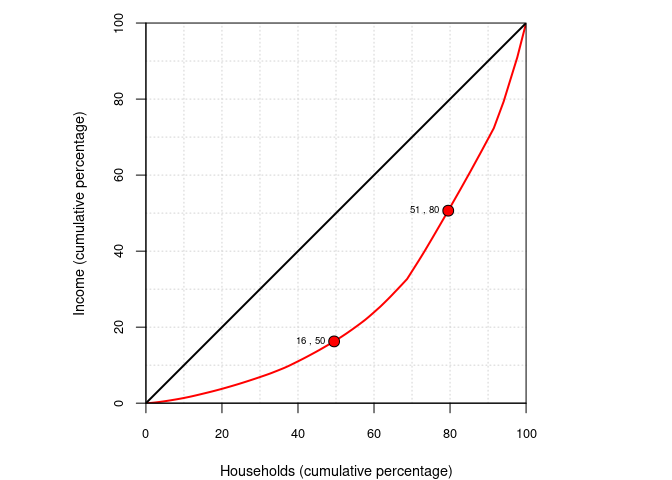The curve depicts on its horizontal axis a defined population – e.g., all households – broken down into deciles and ordered from left to right on the horizontal axis (from the lower tax income per household to the higher). On the vertical axis of the Lorenz curve is shown the cumulative percentage of tax income.

The reading of the plot reveals these following configurations :

• 50 % of the households earns less than 16 % of the total income.
• 50 % of the total income is held by less than 20 % of households.

## 2.2 Territorial deviation

The territorial deviation is now calculated to analyze the position of each municipality as regards to a territorial level of reference: the (EPT) of belonging in this case-study.

The `tdev` function takes in entry the numerator (INC) and the denominator (TH) of the `com` object. The territorial level of belonging in specified with the `key` argument. The territorial relative deviation is returned by the function.

``````# Territorial relative deviation calculation
com\$tdevrel <- tdev(x = com,
var1 = "INC",
var2 = "TH",
type = "rel",
key = "LIBEPT")

# Cartography
# Territorial deviation mapping
mf_map(x = com, var = "tdevrel", type = "choro",
breaks = c(min(com\$tdevrel, na.rm = TRUE), 67, 91, 100, 125, 150,
max(com\$tdevrel, na.rm = TRUE)),
pal = devpal, border = "white", lwd = 0.2,
leg_pos = "topleft", leg_val_rnd = 0,
leg_title = paste0("Deviation to the territorial context",
"\n(100 = EPT average)"))

# Plot EPT layer
mf_map(ept, col = NA, border = "#1A1A19", lwd = 1, add = TRUE)

# Labels to ease comment location
mf_label(x = com[com\$LIBCOM %in% c("Le Raincy", "Rungis", "Sceaux",
"Marnes-la-Coquette") ,],
var = "LIBCOM", cex = 0.6, font = 2, halo = TRUE)

# layout
mf_layout(title = "Territorial deviation - Tax income per households, 2013",
credits = paste0("Sources : GEOFLA® 2015 v2.1, Apur, impots.gouv.fr",
"\nRonan Ysebaert, RIATE, 2021"),
arrow = FALSE)``````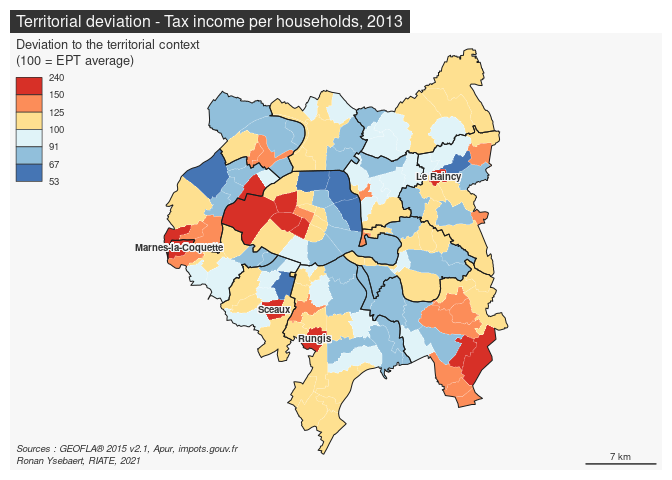This map highlights existing inequalities in each EPT: The strongest differences in relative terms are located in Paris (opposition between the eastern part and the western part of this EPT) and in the Plaine centrale - Haut Val de Marne EPT (opposition between the poorest municipalities located near Paris and the ones located in the periphery). Globally, the richest and the poorest EPT ( Grand Paris Sud Ouest / Plaine Commune and Territoires des Aéroports du Nord Ouest) appear relatively homogeneous statistically. In other EPT, one municipality appears largely above the average of their EPT of belonging. It is namely the case in Grand-Paris-Sud-Ouest (Marnes-la-Coquette), Sud Hauts-de-Seine (Sceaux), Grand Orly Seine Biève (Rungis) or Grand Paris Grand Est (Le Raincy)

Another way to explore characteristics of the territorial deviation consists in analyzing the statistical dispersion (general deviation) by intermediate level (EPT in this case). The best suited graphical representation for this is certainly a boxplot.

The code below takes in entry the general deviation calculated above and the intermediate levels included in the input dataset. It returns a boxplot displaying the statistical parameters (median, mean, 1st and 3rd quartiles, range, minimum and maximum, extraordinary values) allowing to observe the statistical dispersion existing for each intermediate zoning. To ease the interpretation and the synthesis of the plot, boxplots are ordered by the average of each territorial level. Moreover, the width of the bars are proportional to the number of territorial units included in each intermediate zoning.

``````opar <- par(mar = c(4, 4, 1.2, 2))

# Drop geometries
df <- st_set_geometry(com, NULL)

# Reorder EPT according to gdev value
df\$EPT <- with(df, reorder(EPT, gdevrel, mean, na.rm = TRUE))

# Colors management
col <- aggregate(x = df[,"gdevrel"], by = list(LIBEPT = df\$LIBEPT),
FUN = mean)
col <- merge(col, colEpt, by = "LIBEPT")
col <- col[order(col\$x),]
cols <- as.vector(col\$cols)

# Drop inexisting levels
df <- droplevels(df)

# Boxplot
bp <- boxplot(df\$gdevrel ~ df\$EPT, col = cols, ylab = "Global deviation",
xlab = "Territorial deviation", cex.lab = 0.9,
varwidth = TRUE, range = 1.5,  outline = TRUE,  las = 1)

# Horizontal Ablines
abline (h = seq(40, 300, 10), col = "#00000060", lwd = 0.5, lty = 3)

# Plot mean values
xi<- tapply(df\$gdevrel, df\$EPT, mean, na.rm = TRUE)
points(xi, col = "#7C0000", pch = 19)

# Legend for the boxplot
legend("topleft", legend = rev(as.vector(col\$LIBEPT)), pch = 15,
col = rev(as.vector(col\$cols)), cex = 0.8, pt.cex = 1.5)
par(opar)``````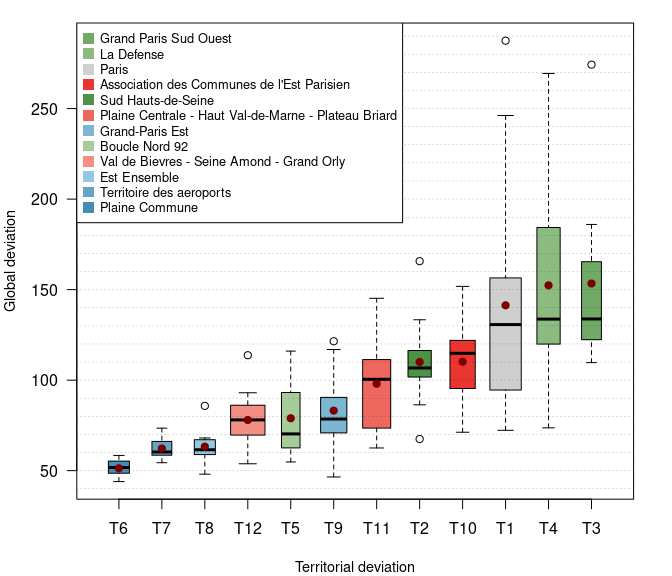This plot highlights the statistical dispersion existing in each EPT. It confirms globally that wealthier the EPT is, larger the statistical differences between the poorest and the wealthiest territorial units are. In that way, Plaine Commune and Territoire des Aéroports (T6 and T7) appear quite homogeneous in a lagging situation. On the opposite, important differences a revealed in Paris (minimum = 71 and maximum = 290) or in La Defense and Grand Paris Sud Ouest.

The boxplot highlights also outliers (dots out of the box) in each territorial context. Most EPT are concerned: wealthiest EPT (Paris, La Défense, Grand Paris Sud Ouest and ACEP, generally characterized by high outliers) and EPT in less favorable situation (Est Ensemble, Grand Paris Est and Sud-Hauts-de-Seine, which include municipalities with include both low and high outliers.

## 2.3 Spatial deviation

The spatial deviation is calculated to position territorial units in a neighborhood context. Several criteria may be considered (geographical distance, time-distance). In this example, each municipality will be compared to the average of contiguous municipalities (contiguity order 1).

The `sdev` function takes in entry the numerator (INC) and the denominator (TH) of the `com` object. The contiguity order 1 is set in the `order` argument.

``````# Spatial relative deviation calculation
com\$sdevrel <- sdev(x = com,
xid = "DEPCOM",
var1 = "INC",
var2 = "TH",
order = 1,
type = "rel")

# Cartography
# Territorial deviation (relative and absolute) cartography
mf_map(x = com, var = "sdevrel", type = "choro",
breaks = c(min(com\$sdevrel, na.rm = TRUE), 67, 91, 100, 125, 150,
max(com\$sdevrel, na.rm = TRUE)),
pal = devpal, border = "white", lwd = 0.2,
leg_pos = "topleft", leg_val_rnd = 0,
leg_title = paste0("Deviation to the spatial context",
"\n(100 = average of the contiguous",
" territorial units - order 1)"))

# Plot EPT
mf_map(ept, col = NA, border = "#1A1A19",lwd = 1, add = T)

# Labels to ease comment location
mf_label(x = com[com\$LIBCOM %in% c("Le Raincy", "Vaucresson", "Sceaux", "Bagneux",
"Marnes-la-Coquette", "Saint-Maur-des-Fosses",
"Puteaux", "Saint-Ouen", "Clichy-sous-Bois",
"Clichy"),],
var = "LIBCOM", cex = 0.6, font = 2, halo = TRUE, overlap = FALSE)

# layout
mf_layout(title = "Spatial deviation - Tax income per households, 2013",
credits = paste0("Sources : GEOFLA® 2015 v2.1, Apur, impots.gouv.fr",
"\nRonan Ysebaert, RIATE, 2021"),
arrow = FALSE)``````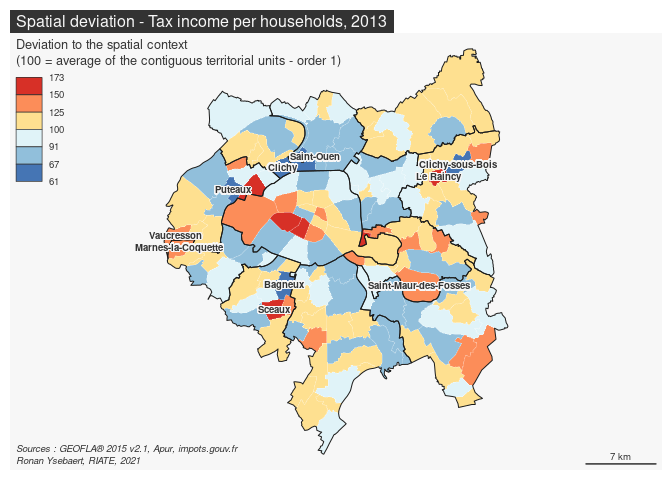This map highlights local discontinuities existing in the study area. Important local statistical gaps appear in several areas: Saint-Mandé and Neuilly-sur-Seine are characterized by the highest score as regards to their respective neighbors (indexes 173 and 156).Some local “bastions” are revealed in Paris suburbs, such as Le Raincy (index 153), Sceaux (150), Vaucresson (143), Marnes-la-Coquette (142) or Saint-Maur-des-Fossés (140).

On the reverse situation, lower index are observed at Clichy-sous-Bois. Clichy, Puteaux, Saint-Ouen and Bagneux. Their average income per household is around 30-40 % below their respective neighborhood.

Spatialized indicators are often subject to spatial dependencies (or interactions), which are even stronger than spatial locations are closer. Autocorrelation measures (Moran, Geary, Lisa indexes) allows estimating the spatial dependence between the values of a same indicator at several locations of a given study area.

The figure displayed below consists in evaluating this spatial autocorrelation by a plot crossing the spatial deviation (Y axis) and global deviation (X axis) values.

This plot provides interesting inputs for answering to basic questions, such as: * Are territorial units in favorable situation in a global context also in the same situation at local level? * Is it possible to detect specific situations as regard to the global trend, meaning the existence of “local bastions” in favorable or lagging situations?

``````opar <- par(cex.lab = 1, cex.axis = 0.75, mar = c(4, 4, 1.2, 2))

# Drop geometries
df <- st_set_geometry(com, NULL)

# Spatial autocorrelation
lm <- summary.lm(lm(sdevrel ~ gdevrel, df))

# Equation
eq <- paste("Spatial Deviation =",
round(lm\$coefficients["gdevrel","Estimate"], digits = 3),
"* (Global Deviation) +",
round(lm\$coefficients["(Intercept)","Estimate"], digits = 3))
rsq <-paste("R-Squared =",
round(summary(lm(sdevrel ~ gdevrel, com ))\$r.squared, digits = 2))

# Color management
df <- merge(df, colEpt, by = "LIBEPT")

# Plot spatial autocorrelation
plot(df\$gdevrel, df\$sdevrel,
ylab = "Local deviation",
ylim = c(50,260),
xlab = "Global deviation",
xlim = c(50,260),
pch = 20,
col = as.vector(df\$col),
asp = 1)
abline((lm(df\$sdevrel ~ df\$gdevrel)), col = "red", lwd =1)

# Specify linear regression formula and R-Squared of the spatial autocorrelation
text(110,60, pos = 4, cex = 0.7, labels = eq)
text(110,55, pos = 4, cex = 0.7, labels = rsq)

abline (h = seq(40,290,10), col = "gray70", lwd = 0.25, lty = 3)
abline (h = seq(50,250,50), col = "gray0", lwd = 1, lty = 1)
abline (v = seq(40,290,10), col = "gray70", lwd = 0.25, lty = 3)
abline (v = seq(50,250,50), col = "gray0", lwd = 1, lty = 1)

# Legend for territorial level
legend("topleft", legend = rev(as.vector(colEpt\$LIBEPT)), pch = 15,
col = rev(as.vector(colEpt\$cols)), cex = 0.6, pt.cex = 1.5)
par(opar)``````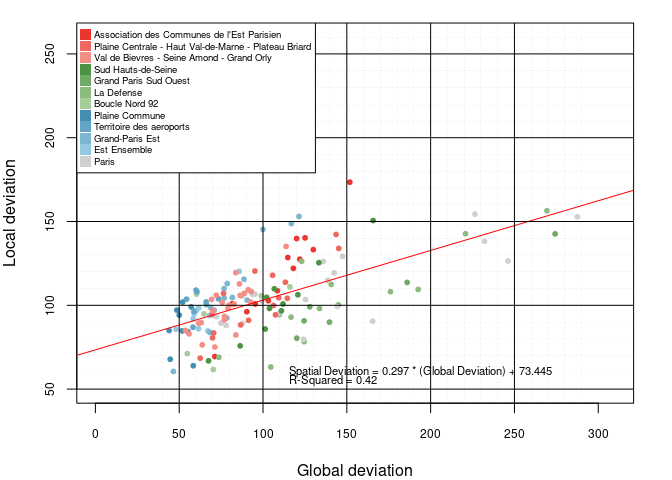The output of the linear model of spatial autocorrelation reveals that the hypothesis of independence is rejected at a probability below than 0.0001. It means that, “everything is related to everything else, but near things are related than distant things” (Tobler, 1970). However, the R-squared of the relation (0.42) suggests that this statistical relation includes outliers very far from the linear regression.

This chart can be modified for analyzing outliers specifically. The code below computes the statistical residuals of the spatial autocorrelation calculated above. Then the residuals are standardized. Finally, a plot is built to display and label significant residuals.

``````opar <- par(cex.lab = 1, cex.axis = 0.75, mar = c(4, 4, 2, 2))

# Standardized residual calculation
lm <- lm(sdevrel ~ gdevrel, df)
df\$res <- rstandard(lm)

#risk alpha (0.1 usually)
alpha <- 0.055

# Calculation of the threshold using T-Student at (n-p-1) degrees of freedom
thr <- qt(1 - alpha / 2, nrow(com) - 1)

# Plot residuals
plot(df\$sdevrel, df\$res,
xlab = "Local deviation", cex.lab = 0.8,
ylim = c(-3.5, 3.5),
xlim = c(40, 200),
ylab = "Standardized residuals of spatial autocorrelation",
cex.lab = 0.8,
cex.axis = 0.8,
pch = 20,
col = as.vector(df\$cols))

abline(h = - thr, col = "red")
abline(h = + thr, col = "red")
abline(h = 0, col = "red")

# Detecting exceptional values and labeling them on the plot
ab <- df[df\$res < -thr | df\$res > thr,]

# Plot residual labels
text(x = ab[,"sdevrel"], y = ab[,"res"], ab[,"LIBCOM"], cex = 0.5, pos = 4)

abline (v = seq(50, 200, 10), col = "gray70", lwd = 0.25, lty = 3)
abline (v = seq(50, 200, 50), col = "gray0", lwd = 1, lty = 1)

# Plot the legend (territorial zoning)
legend("topleft", legend = rev(as.vector(colEpt\$LIBEPT)), pch = 15,
col = rev(as.vector(colEpt\$cols)), cex = 0.6, pt.cex = 1.5)
par(opar)``````